Tamilnadu State Board New Syllabus Samacheer Kalvi 12th Maths Guide Pdf Chapter 12 Discrete Mathematics Ex 12.2 Text Book Back Questions and Answers, Notes.

## Tamilnadu Samacheer Kalvi 12th Maths Solutions Chapter 12 Discrete Mathematics Ex 12.2

Question 1.
Let p : Jupiter is a planet and q: India is an island be any two simple statements. Give verbal sentence describing each of the following statements.
(i) ¬P
(ii) P ∧ ¬q
(iii) ¬p v q
(iv) p → ¬q
(v) p ↔ q
Solution:
p: Jupiter is a planet
q: India is an island
(i) ¬P : Jupiter is not a planet
(ii) P ∧ ¬q : Jupiter is a planet and India is not an island.
(iii) ¬p v q : Jupiter is not a planet or India is an island
(iv) p → ¬q : Jupiter is a planet then India is not an island
(v) p ↔ q : Jupiter is a planet if and only if India is an islandQuestion 2.
Write each of the following sentences in symbolic form using statement variables p and q.
(i) 19 is not a prime number and all the angles of a triangle are equal.
(ii) 19 is a prime number or all the angles of a triangle are not equal.
(iii) 19 is a prime number and all the angles of a triangle are equal.
(iv) 19 is not a prime number.
Solution:
Let p : 19 is a prime number
q : All the angles of a triangle are equal
(i) 19 is not a prime number and all the angles of a triangle are equal ⇒ ¬p ∧ q
(ii) 19 is a prime number or all the angles of a triangle are not equal ⇒ p v ¬q
(iii) 19 is a prime number and all the angles of a triangle are equal ⇒ p ∧ q
(iv) 19 is not a prime number ⇒ ¬p

Question 3.
Determine the truth value of each of the following statements
(i) If 6 + 2 = 5, then the milk is white.
(ii) China is in Europe dr √3 is art integer
(iii) It is not true that 5 + 5 = 9 or Earth is a planet.
(iv) 11 is a prime number and all the sides of a rectangle are equal.
Solution:
(i) If 6 + 2 = 5, then milk is white,
p: 6 + 2 = 5(F)
q: Milk is white (T)
∴ p → q is having the truth value T

(ii) China is in Europe or √3 is an integer
p: China is in Europe (F)
q: √3 is an integer (F)
∴ p v q is having the truth value F

(iii) It is not true that 5 + 5 = 9 or Earth is a planet
p: 5 + 5 = 9 (F)
q: Earth is a planet (T)
∴ ¬p v q is having the truth value T

(iv) 11 is a prime number and all the sides of a rectangle are equal.
p : 11 is a prime number (T)
q: All the sides of a rectangle are equal (F)
∴ p ∧ q is having the truth value FQuestion 4.
Which one of the following sentences is a proposition?

1. 4 + 7 = 12
2. What are you doing?
3. 3ⁿ ≤ 81, n ∈ N
4. Peacock is our national bird.
5. How tall this mountain is!

Solution:

1. is a proposition
2. not a proposition
3. is a proposition
4. is a proposition
5. not a proposition

Question 5.
Write the converse, inverse, and contrapositive of each of the following implication.
(i) If x and y are numbers such that x = y, then x² = y²
(ii) If a quadrilateral is a square then it is a rectangle.
Solution:
(i) Converse: If x and y are numbers such that x2 = y2 then x = y.
Inverse: If x and y are numbers such that x ≠ y then x2 ≠ y2.
Contrapositive: If x and v are numbers such that x2 ≠ y2 then x ≠ y.

(ii) Converse: If a quadrilateral is a rectangle then it is a square.
Inverse: If a quadrilateral is not a square then it is not a rectangle.
Contrapositive: If a quadrilateral is not a rectangle then it is not a square.Question 6.
Construct the truth table for the following statements.
(i) ¬P ∧ ¬q
(ii) ¬(P ∧ ¬q)
(iii) (p v q) v ¬q
(iv) (¬p → r) ∧ (p ↔ q)
Solution:
(i) ¬P ∧ ¬q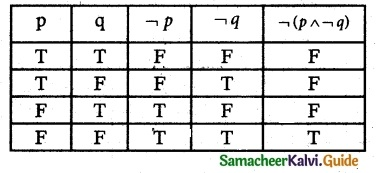(ii) ¬(P ∧ ¬q)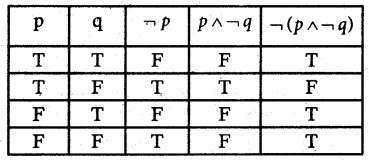(iii) (p v q) v ¬q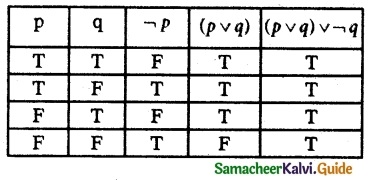(iv) (¬p → r) ∧ (p ↔ q)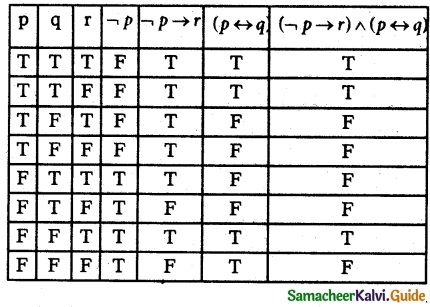Question 7.
Verify whether the following compound propositions are tautologies or contradictions or contingency.
(i) (P ∧ q) ∧¬ (p v q)
(ii) ((P v q) ∧¬p) → q
(iii) (p → q) ↔ (¬p → q)
(iv) ((p → q) ∧ (q → r)) → (p → r)
Solution:
(i) (P ∧ q) ∧¬ (p v q)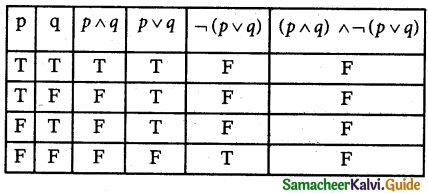The entries in the last column are only F.
∴ The given statement is a contradiction

(ii) ((P v q) ∧¬p) → q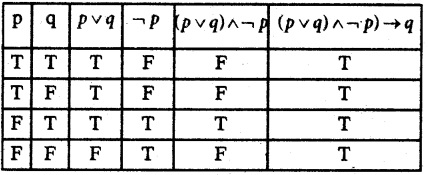The entries in the last column are only T.
∴ The given statement is a Tautology

(iii) (p → q) ↔ (¬p → q)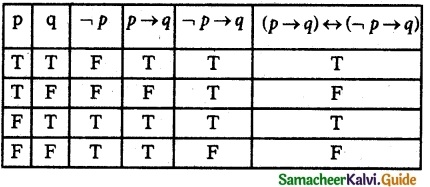The entries in the last column are a combination of T and F.
∴ The given statement is a contingency.

(iv) ((p → q) ∧ (q → r)) → (p → r)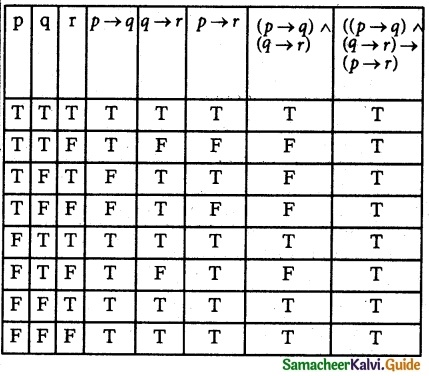All the entries in the last column are only T.
∴ The given statement is a tautology.Question 8.
Show that (i) ¬(p ∧ q) ≡ ¬P v ¬q
(ii) ¬(p → q) ≡ p ∧¬q
Solution:
(i) ¬(p ∧ q) ≡ ¬P v ¬q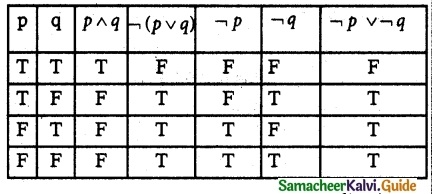The entries in the columns corresponding to ¬(p ∧ q) and ¬P v ¬q are identical and hence they are equivalent.

(ii) ¬(p → q) ≡ p ∧ ¬q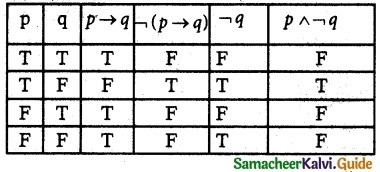The entries in the columns corresponding to ¬(p → q) and p ∧ ¬q are identical and hence they are equivalent.

Question 9.
Prove that q → p ≡ ¬p → ¬q
Solution:
q → p ≡ ¬p → ¬q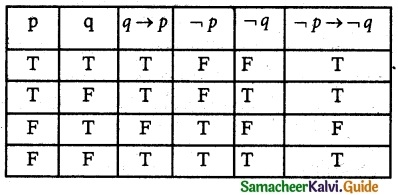The entries in the columns corresponding to q → p and ¬p → ¬q are identical and hence they are equivalent.
∴ q → q = ¬p → ¬q
Hence proved.Question 10.
Show that p → q and q → p are not equivalent
Solution: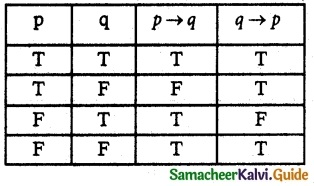From the table, it is clear that
p → q ≠ q → P

Question 11.
Show that ¬(p ↔ q) ≡ p ↔ ¬q
Solution: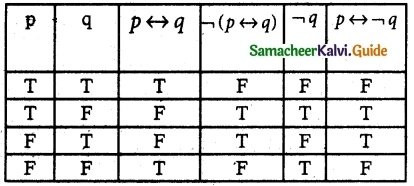From the table, it is clear that
¬(p ↔ q) ≡ p ↔ ¬qQuestion 12.
Check whether the statement p → (q → p) is a tautology or a contradiction without using the truth table.
Solution:
P → (q → p)
≡ P → (¬q v p) [∵ implication law]
≡ ¬p v (¬q v p) [∵ implication law]
≡ ¬p v (p v ¬q) [∵ Commutative law]
≡ (¬p V p) v (¬p v ¬q) [∵ Distribution law]
≡ T v ¬(p ∧ q) ≡ T [Tautology]
Hence p → (q → p) is a Tautology.

Question 13.
Using the truth table check whether the statements ¬(p v q) v (¬p ∧ q) and ¬P are logically equivalent.
Solution: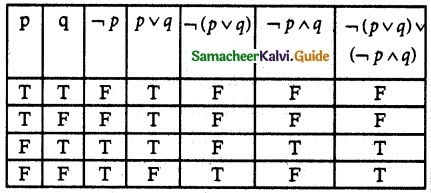From the table, it is clear that ¬P and
¬(p v q) v (¬p ∧ q) are logically equivalent
i.e. ¬(p v q) v (¬p ∧ q) ≡ ¬p

Question 14.
Prove p → (q → r) ≡ (p ∧ q) → r without using the truth table.
Solution:
P → (q → r)
≡ P → (¬q v r) [∵ implication law]
≡ ¬p v (¬q v r) [∵ implication law]
≡ (¬p v ¬q) v r [∵ Associative law]
≡ ¬(p ∧ p) v r [∵ DeMorgan’s law]
≡ (p ∧ q) → r ≡ T [∵ implication law]
Hence Proved.Question 15.
Prove that p → (¬q v r) ≡ ¬p v (¬q v r) using truth table.
Solution: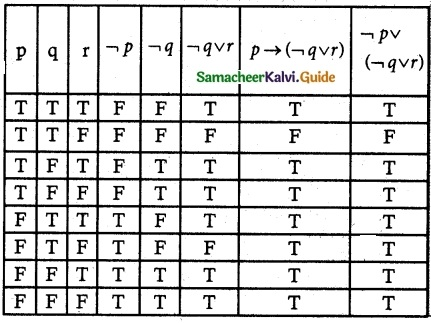From the table, it is clear that the column of p → (¬q v r) and ¬p v (¬q v r) are identical.
∴ p → (¬q v r) ≡ ¬p v (¬q v r)
Hence proved.